# Research on Human Action Recognition Algorithm Based on Video Image

Le Gao1, Yongjie Huang1, Wanting Zhang1, Tian Yang1* and Jin Hong2

1Wuyi University, China

2The Chinese University of Hong Kong, China

Received Date:April 14, 2021;  Published Date: April 28, 2021

#### Abstract

Human Action recognition is one of the research hotspot in the field of computer vision and artificial intelligence. It can usually be applied in many fields such as virtual reality, intelligent pension, medical monitoring, child monitoring, public safety and business management. Due to the complexity and variability of human movements, it is difficult and challenging to use computers to accurately identify actions. Therefore, it is of great significance to study the algorithm of motion recognition for video images. In the study of motion recognition algorithm, RGB data are used as input, and Slowfast Network and I3D algorithm models are used for training respectively. Experimental results show that the training accuracy of SlowFast algorithm is 96.66%. The training accuracy of I3D algorithm is as high as 99.16%, and both algorithms can accurately identify human movements. This study confirms that the human motion recognition technology and algorithm will have a wide range of application scenarios in the future. After solving the technical problems of low recognition rate, the recognition algorithm and technology will be widely promoted and applied in various fields.

Keywords: Action Recognition; Slow Fast Algorithm; I3D Algorithm; RGB

#### Introduction

Human motion recognition can be used in many fields such as virtual reality, intelligent elderly care, medical monitoring, child monitoring, public safety, and business management . However, human movements are complex and changeable, and there are certain difficulties and challenges in using computers to accurately identify them. Therefore, the research on the algorithm of motion recognition of video images is of great significance [2-5]. Video based human motion recognition data sources can be mainly divided into two categories [6,7]: one is RGB data, and the other is RGB-D data. RGB data contains a wider range of appearance information than RGB-D data. RGB data has richer colors, clearer image textures, and more attention to detail. However, it is more difficult to obtain RGB data. For example, when collecting RGB data, the data are more likely to be affected by external factors, such as environment, illumination, and clothing. RGB-D data is not affected by these factors and can obtain reliable human contour and skeleton information. These two types of data sources have their own advantages and disadvantages and can be used in different scenarios. In recent years, RGB data source has become a research hotspot in the field of motion recognition, and RGB data can be depicted more accurately in the process of human working recognition. At present, a large number of scholars have carried out a large number of research in the field of deep learning based on video and image and made great progress in this field [8-10]. However, the effective use of machines and computers to recognize human movements is a challenging task, which requires continuous exploration by researchers. In the following, human motion recognition algorithms and techniques based on RGB data are discussed.

#### Overview of Video Image Recognition Algorithms

##### A traditional image recognition algorithm-convolutional 3D

In the study of video images, one of the commonly used algorithms is Convolutional 3D (C3D). Since video data has one more time feature than picture data, more in-depth 3D convolutional neural network should be used in the practice of video data, instead of using 2D convolutional neural network as in the case of picture data. In the study of 2D image and 3D image, the output of 2D convolution is a 2D feature map, while the output of 3D convolution is still a 3D feature ma/formed in space, while the convolution and pooling of the 3D convolutional neural network are operated in space and time. If the size of a video data is a×b×c×d, where a is the number of channels (RGB images are generally 3), b is the number of frames of the video, and c and d are the height and width of each frame respectively. The convolutional kernel and pooled kernel of 3D convolutional neural network are also 3D, that is, extending a dimension from 2D convolutional neural network, so the kernel is e×f×f, where E is the time depth of the kernel, and f×f is the space size of the kernel . Therefore, compared with 2D convolutional neural network, 3D convolutional neural network has better modeling ability of spatial and temporal information, and 3D convolutional neural network is more suitable for processing video data. Figure 1 shows the C3D image recognition model through convolution operation (Figure1).##### Long-term recurrent convolutional network (LRCN)

In the C3D image recognition method, 2D image convolution is converted into 3D image convolution, so that the trained model only has simple time characteristics. In order to solve the problem of gradient explosion or gradient disappearance in longterm memory, the long-term Recurrent Convolutional Network (LRCN) algorithm can be adopted. LRCN algorithm combines the advantages of Recurrent Neural Network (RNN) algorithm and Long Short-Term Memory (LSTM) algorithm, which can extract image features effectively, and then transmit image feature modules to the next module in an orderly and complete manner. In addition, the problem of long-term memory is maintained and the problem of spatial and temporal dimensions of images is well handled. As shown in Figure 2, it is an LRCN algorithm model, which extracts n frames from the research video images, processes them through the convolutional network, and then connects them to the LSTM layer. In the LSTM layer, the predicted categories of n frames are made, and finally the predicted results are calculated according to the weight (Figure 2).##### Slowfast network algorithm

The SlowFast Network algorithm is derived from the retinal nervous system of primates. In primate retinal nerves, about 80 percent of the cells operate at low frequencies, used to process spatial detail and color, but are insensitive to rapid changes in things. And about 20 percent of the cells operate at high frequencies to deal with rapid changes. There are two paths in SlowFast Network, one is Slow path and the other is Fast path. The calculation cost of Slow path is about 4 times of that of Fast path. The Fast path takes α times as many frames as the Slow path, where α is usually set to 8. Set the time step with τ=α, that is, when Slow collects T frames, Fast path collects αT frames. The number of channels in a Slow path is typically β times the number of channels in a Fast path, and β is usually set to 8. Table 1 shows SlowFast Network algorithm model. In this model, Slow path and Fast path will first extract image characteristic values through convolutional neural Network respectively, and then connect the Slow path and Fast path together through the Resnet model group and finally through the global average pooling layer to output the full connection. In addition, in this model, the Slow path will obtain the data calculated by the Fast path through the lateral connection, calculate with the data of the Slow path itself, and send the data processed by the Fast path to the Slow path through the convolution operation (Table 1).

Table 1: Slow fast Network Algorithm Model 1.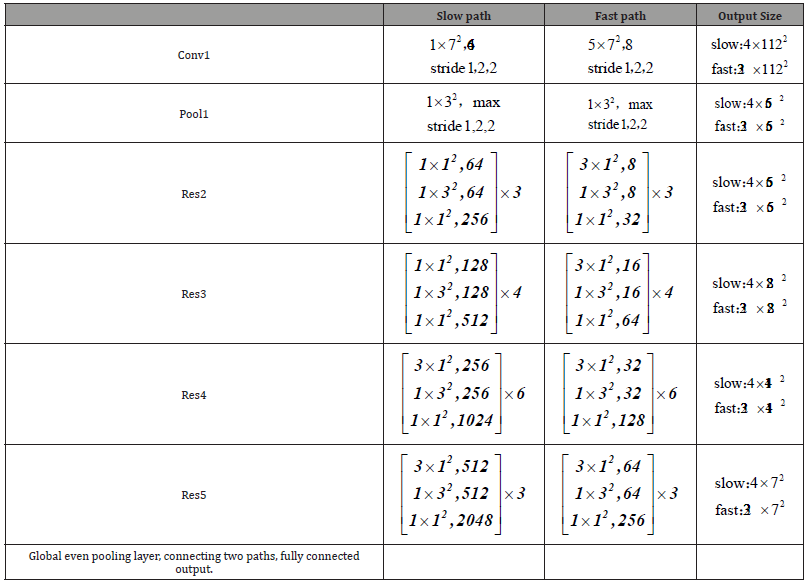Table 2: Comparison of Experimental Results.##### Inflated 3D convnets algorithm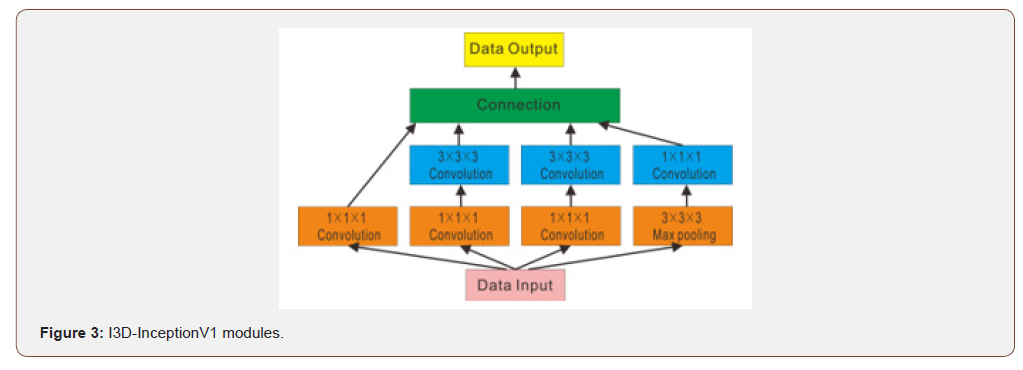Inflated 3D ConvNets model is expanded from 2DCNN Invection-V1. I3D can convert from 2D to 3D using parameters pretrained on an ImageNet, and apply parameters learned using a 2D filter to a 3D filter. The experimental results show that the I3D model achieves very good results on all standard data sets. Figure 3 shows the I3D-InceptionV1 module. The input image data is convoluted and parallel calculated, and then the calculated results are connected and output. Figure 5 is an upgrade on the basis of the i3D-InceptionV1 module. The input video image is first processed through multiple convolution layers and two or more Inception modules, then processed through the global average pooling layer and finally entered into the full connection layer (Figure 3 & 4).

#### Data Preprocessing

The experiments and research in this paper are carried out in the Institute of Bluedon Information Security, Wuyi University. The computer operating system is Linux, two GPU graphics cards are used, the model is GTX3090, and data set BL004 is used for training and testing. The video images of BL004 data set are from iQIYI and Tencent video platforms, and it contains 100 video data in total, including 30 groups of actions, and each type of action is composed of 10 people’s videos.

##### Experiment of slowfast network algorithm

Before the experiment began, the first step was to preprocess the BL004 data set with a unified resolution of 256×256 and the length of each video was cut into 5 seconds. Step 2: Divide the video data into four groups of actions: sitting, falling, walking and standing up. Put the four groups of actions into four folders respectively, and assign labels to each group of actions, that is, 0,1,2,3 respectively represent sitting, falling, walking and standing up; Step 3: Write a program language to extract the required frames of the experimental test from each video; Step 4: Perform the following processing for each frame image: a. Corner clipping and multi-scale clipping to obtain a 224×224 image. B. Make a random horizontal flip with a probability of 0.5. C. Normalization of values. D. Attach a specific label to each video. Figure 5 is the effect diagram after data processing, Figure 6abc is the data before data processing, and Figure 5ABC is the data after data processing. After the data is processed, every frame is cropped, flipped, and the values range from 0-255, to 0-1.

#### Experiment

After comprehensive analysis, this study mainly uses Slowfast Network algorithm and I3D algorithm to compare and discuss.In the Slowfast Network algorithm model, the Resnet50 module contains three convolution layers: 1×1, 3×3 and 1×1 spatial dimension convolution layer (Figure 5).

The 1×1 convolution kernel is to change the number of channels, and the 3×3 convolution kernel is to change the dimension size of the step, and the eigenvalue can be further learned. In the time dimension, the filling value and stride size can be changed to ensure that the time dimension size remains the same. The algorithm goes through the normalization layer after each convolution, and finally activates the function through rule. In the side connection convolution layer in the algorithm model, a 5×1×1 convolution kernel is first passed with a step of 8×1×1 and filled with 2×0×0. Then it goes through the normalization layer and activates the function through the Rule.

The specific steps of the experiment are as follows:

• Step 1: Save the preprocessed pictures into the library. Slow path takes 4 frames, Fast path takes 32 frames, each frame is 224×224 feature image size, the number of channels is 3. The size of input data for Slow path is 3×4×224×224, and the size of input data for Fast path is 3×32×224×224, which are then input into the neural network for training.

• Step 2: Convolved the trained data through the convolution layer, then through the normalization layer and rule activation function, and finally through the maximum pooling layer. The size of output data of the Fast path is 8×32×56×56, and the output results of the Fast path are transmitted to the Slow path through the side connection, and the size of output data through the Slow path is 80×4×56×56.

• Step 3: Transfer the video data of the previous step through three Resnet50 modules, and then the output data size of FAST path is 32×32×56×56. Transfer the output results of FAST to the Slow path through the side connection, and finally the output data size of the Slow path is 320×4×56×56.

• Step 4: Pass the video data of the previous step through four Resnet50 modules. The output data size of the Fast path is 64×32×28×28. The output result of FAST is transmitted to the Slow path through the side connection, and the final output data size of the Slow path is 640×4×28×28.

• Step 5: Pass the video data of the previous step through three Resnet50 modules. The output data size of the Fast path is 128×32×14×14. The output result of FAST is transmitted to the Slow path through the side connection, and the final output data size of the Slow path is 1280×4×14×14.

• Step 6: Pass the video data of the previous step through three Resnet50 modules. The output data size of the Fast path is 256×32×7×7. The output result of FAST is transmitted to the Slow path through the side connection, and the final output data size of the Slow path is 2048×4×112×112.

• Step 7: Put the output results of both FAST path and SLOW path through the global average maximum pooling layer.

• Step 8: Connect the output results of the Fast path and the Slow path and discard them with a probability of 0.5.

• Step 9: Put the result of the previous step through the full connection layer with the number of output channels being 4 and use softmax to output the result (Figure 6).The experimental results of the training set of Slowfast Network experiment are shown in Figure 7. With the increase of training times, the entropy loss is decreased, and the accuracy of prediction is increased. The entropy loss in Figure 6a is stable at about 0.11. The accuracy of the predicted results in Figure 6b was above 95%, with the highest reaching 96.66%. Figure 7 shows the experimental results of the test set. Its accuracy stability is not high, but the accuracy rate is improving in general, and the final accuracy rate reaches more than 80%. In summary, the experimental results of the training set and the test set show that the loss entropy and prediction accuracy of the training set are gradually stable after 40 times, and the accuracy of the test set is also gradually stable after 55 times. The accuracy of the training set was 96.66%, and the accuracy of the test set was more than 80%. The experimental results show that the model trained by this algorithm has a more accurate ability to recognize the action data and can effectively classify different action behaviors (Figure 7).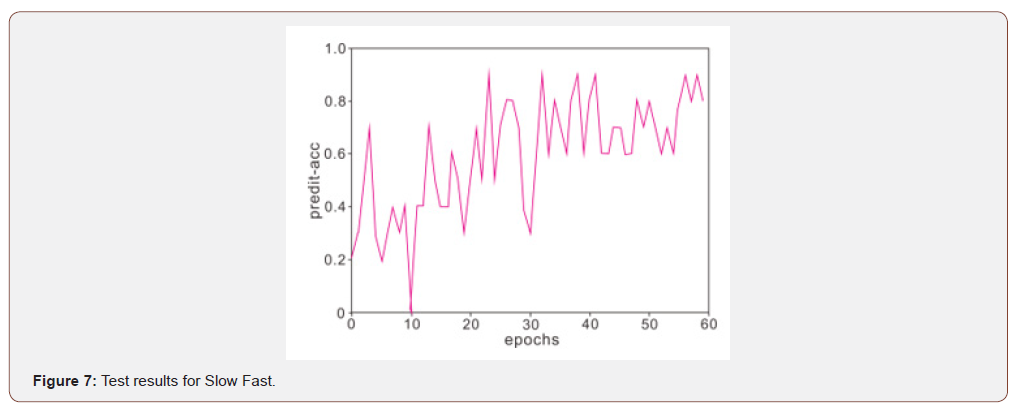##### I3D algorithm experiment

To better verify the performance of the motion recognition algorithm, the BL004 data set in this study was experimented with I3D algorithm, and the reliability of the I3D algorithm and the Slowfast Network algorithm were compared and analyzed. Fourstep parallel computation is carried out in the InceptionV1 module of the I3D algorithm model:

• The first part passes through the convolution layer whose convolution kernel is 1.

• The second part passes through the convolution kernel whose convolution kernel is 1, and then through the convolution layer whose convolution kernel is 3.

• The third part passes through the convolution kernel whose convolution kernel is 1, and then passes through the convolution layer whose convolution kernel is 3.

• In the fourth part, the maximum pooling layer with size 3, step 1 and filling 1 is passed, and the convolution kernel with convolution kernel 1 is passed; Finally, all the output channels of the above 4 parts are merged.

The specific experimental steps of I3D algorithm are as follows:

• Step 1: 20 frames are taken from the pre-processed video image, and the size of each frame’s feature image is 224×224. The 20×224×224 data was taken as the input data, the number of channels was defined as 3, and the data was input into the neural network for training.

• Step 2: Spread the trained data to the convolution layer with convolution kernel 7, step length 2 and fill 3. The output data size is 10×112×112 and the number of channels is 64. The output data were then maximized by pooling with size (1,3,3), step (1,2,2) and filling (0,1,1), and 64 characteristic video images of 10×56×56 were obtained.

• Step 3: Propagate the video image in the previous step to the convolution layer with convolution kernel 1 for processing. Then the second processing is carried out through the convolution layer whose convolution kernel is 3 and filled with 1. The output data is then maximized by pooling with size (1,3,3), step (1,2,2), and fill (0,1,1). Finally, 192 10×28×28 feature videos were obtained.

• Step 4: Transfer the feature video of the previous step through two InceptionV1 modules and output the feature video of 10×28×28.

• Step 5: Transfer the feature video in the previous step to the maximum pooling layer, the size of the pooling layer is 3, the step is 2, and the filling is 1. In this step, 480 feature videos of 5×14×14 will be output.

• Step 6: Transfer the feature video of the previous step through five InceptionV1 modules, and output 832 feature videos of 5×14×14.

• Step 7: Transfer the feature video in the previous step to the maximum pooling layer, and the size of the pooling layer is 2 and the step length is 2. This step will output 832 2×7×7 feature videos.

• Step 8: Transfer the feature video in the previous step through two InceptionV1 modules, and output 1024 feature videos of 2×7×7.

• Step 9: Propagate the feature video in the previous step to the global average pooling layer and discard it with a probability of 0.5.

• Step 10: The above data is output through the full connection layer with 4 channels, and Softmax is used for output.

The experimental results of the training set of the I3D experiment are shown in Figure 8. With the increase of the training times, the entropy loss is continuously reduced, and the accuracy of the prediction is continuously increased. The entropy loss in Figure 8a is stable at about 0.015. The accuracy of the predicted results in Fig. 9b was more than 95%, and the highest was 99.16%. Figure 9 shows the experimental results of the test set. Its accuracy stability is not high, but the overall accuracy rate is constantly improving, and the final accuracy rate reaches more than 90%. In summary, the experimental results of the training set and the test set show that the loss entropy of the training set and the prediction accuracy gradually leveled off after 25 times, and the accuracy of the test set also leveled off after 25 times. The accuracy of the training set is 99.16%, and the accuracy of the test set is over 90%. The experimental results show that the model trained by this algorithm has a more accurate ability to recognize the action data and can effectively classify different action behaviors.

#### Analysis and Discussion

In order to improve the accuracy of human action recognition in complex scenes, a variety of algorithms are studied based on public video image data in this paper. Experiments show that the accuracy of both SlowFast method and I3D method can reach more than 96% on human motion data, and the system can recognize human motion accurately, which verifies the feasibility and effectiveness of the proposed algorithms. As shown in Table 2, the entropy loss of calculation results of SlowFast is 10 times that of I3D, and the accuracy of training set is about 2.5% lower than that of I3D, and the accuracy of test set is about 10% lower. In addition, the calculation time of SlowFast is about 1.7 times that of I3D. In conclusion, the experimental results of I3D on this training set are better than that of Slow Fast.

#### Conclusion

The human motion recognition technology and algorithm will have a wide range of application scenarios in the future. After solving the technical problems of low recognition rate, the recognition algorithm and technology will be widely promoted and applied in various fields (Figure 8 & 9).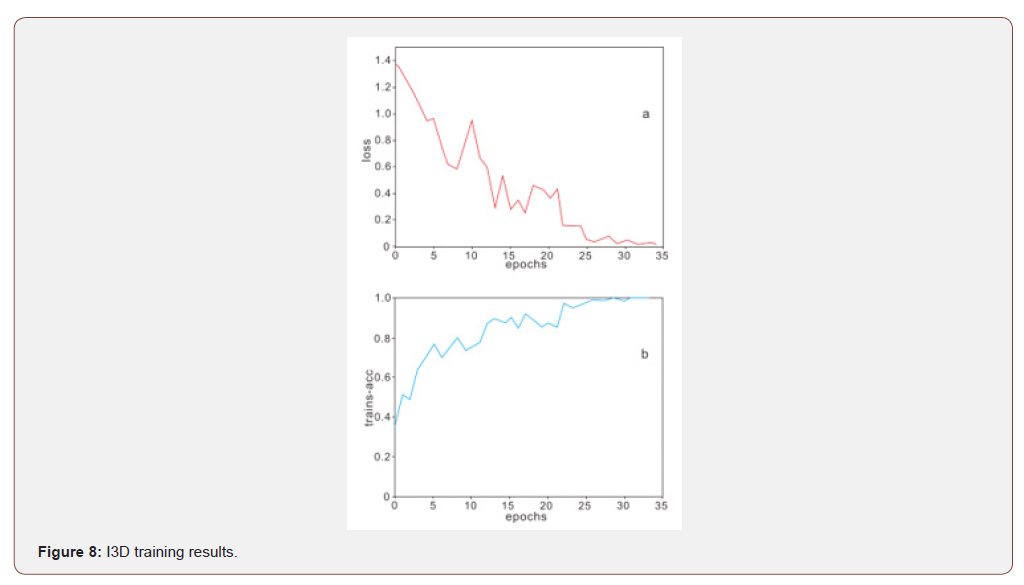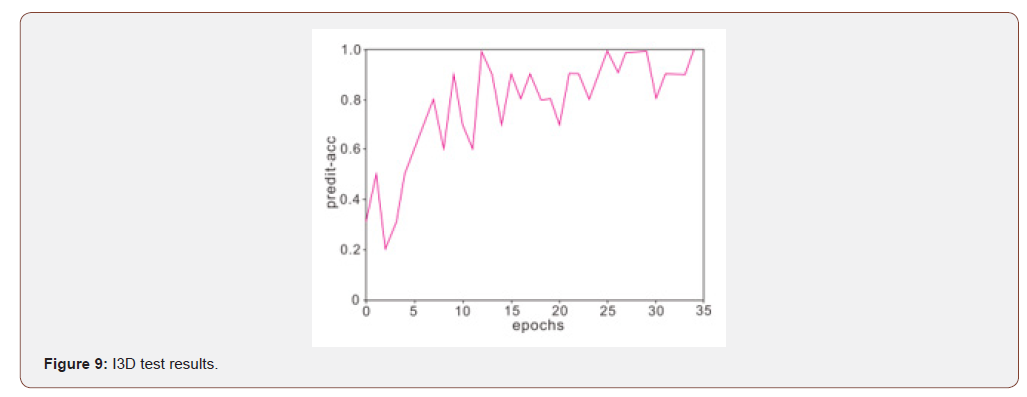#### Acknowledgement

This project is supported by “Natural Science Foundation of Guangdong Province:18zxxt52” “Guangdong Provincial Teaching Reform Project: GDJX2020009” and “Teaching Reform Project of Wuyi University:JX2020052”.

#### Conflict of Interest

Authors declare no conflict of interest.

Article Details
Citation
Keywords
Scroll to
Scroll to Top